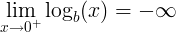# Logarithm of Zero

What is the logarithm of zero? Why log(0) is not defined.

The real logarithmic function logb(x) is defined only for x>0.

We can't find a number x, so the base b raised to the power of x is equal to zero:

b x = 0 , x does not exist

So the base b logarithm of zero is not defined.

logb(0) is not defined

For example the base 10 logarithm of 0 is not defined:

log10(0) is not defined

The limit of the base b logarithm of x, when x approaches zero from the positive side (0+), is minus infinity:Logarithm of one ►